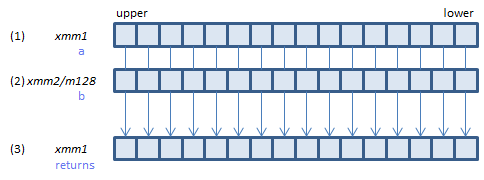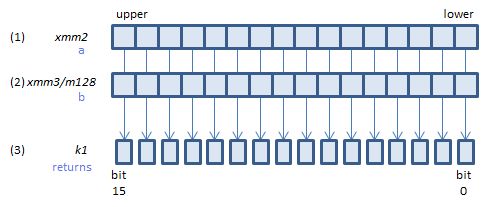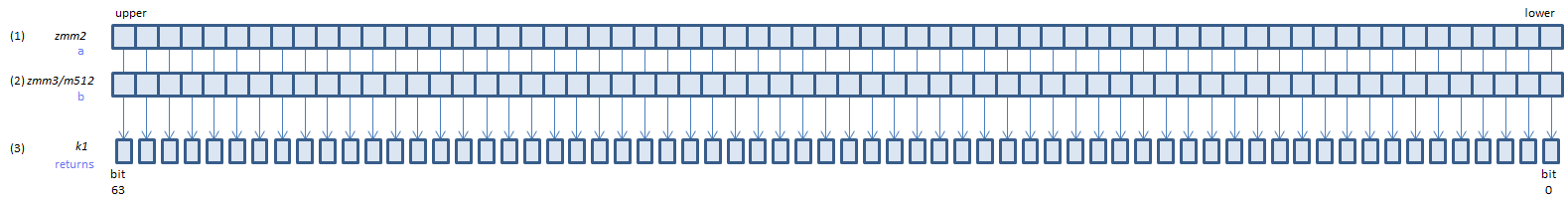﻿ pcmpeqb

## PCMPEQB - Packed CoMPare EQual Byte

PCMPEQB xmm1, xmm2/m128    (S2
__m128i _mm_cmpeq_epi8(__m128i a, __m128i b)For each BYTE, if (1) == (2) set 1, else set 0, to all bits in the corresponding BYTE of (3).
VPCMPEQB xmm1, xmm2, xmm3/m128    (V1
__m128i _mm_cmpeq_epi8(__m128i a, __m128i b)For each BYTE, if (1) == (2) set 1, else set 0, to all bits in the corresponding BYTE of (3).
VPCMPEQB ymm1, ymm2, ymm3/m256    (V2
__m256i _mm256_cmpeq_epi8(__m256i a, __m256i b)For each BYTE, if (1) == (2) set 1, else set 0, to all bits in the corresponding BYTE of (3).
VPCMPEQB k1{k2}, xmm2, xmm3/m128    (V5+BW+VLFor each BYTE, if (1) == (2) set 1, else set 0, to the corresponding bit of (3).
If k2 bit is 0, the comparison is not done and the corresponding bit of (3) is set to zero. Upper bits of (3) are zero cleared.
VPCMPEQB k1{k2}, ymm2, ymm3/m256    (V5+BW+VL